AP State Syllabus AP Board 9th Class Physical Science Solutions Chapter 2 Laws of Motion Textbook Questions and Answers.

## AP State Syllabus 9th Class Physical Science Solutions 2nd Lesson Laws of Motion

### 9th Class Physical Science 2nd Lesson Laws of Motion Textbook Questions and Answers

Question 1.
Explain the reasons for the following. (AS 1)
a) When a carpet is beaten with a stick, dust comes out of it.

1. The dust particles in the carpet are at rest.
2. When the carpet is beaten with a stick, the state of rest of the dust particles is disturbed.
3. Due to inertia, the dust particles comes out.

b) Luggage kept on the roof of a bus is tied with a rope.

1. Luggage kept on the roof of a bus is in the state of rest.
2. As the bus moves, the luggage also moves with a velocity equal to the velocity of the bus.
3. If the bus suddenly stops, the luggage resists to change its state of motion.
4. Hence due to inertia it will fall down.
5. To avoid this, the luggage is tied with a rope.

c) A pace bowler in cricket runs in from a long distance before he bowls.

1. When he runs in from a long distance, he gains momentum of inertia.
2. Due to this larger inertia, larger force is applied in a short interval of time.
3. Hence the momentum will be more.Question 2.
Two objects have masses 8 kg and 25 kg. Which one has more inertia? Why? (AS 1)

1. The object with mass 25 kg has more inertia.
2. The resistance to change the state of object will be more for a body of larger mass.

Question 3.
Keep a small rectangular shaped piece of paper on the edge of a table and place an old five rupee coin on its surface vertically as shown in the figure below. Now give a quick push to the paper with your finger. How do you explain inertia with this experiment?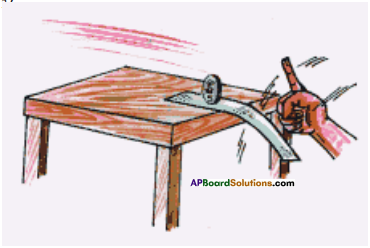1. The coin and the paper are in inertia of rest.
2. When we give a quick push to the paper, paper comes to inertia of motion and the coin remains in its original state i.e., inertia of rest.
3. As a result, the paper will come out and the coin remains on the table without changing its position.

Question 4.
If a car is travelling westwards with a.constant speed of 20 m/s, what is the resultant force acting on it? (AS 1, AS 7)

1. A car is moving with a constant speed.
2. Hence the net force on the car is zero both in horizontal and vertical directions.Question 5.
What is the momentum of a 6.0 kg bowling ball with a velocity of 2.2 m/s? (AS 1)
Mass of the ball (m) = 6.0 kg
Velocity of the ball (v) = 2.2 m/s
Momentum (p) = nv = 6.0 kg × 2.2 m/s = 13.2 kg m/s (or) 13.2 N-s

Question 6.
Two people push a car for 3 sec, with a combined net force of 200 N. (AS 1)
a) Calculate the impulse provided to the car.
Fnet = 200 N ; ∆t =3 sec
Impulse ∆p = Fnet. ∆t = 200 × 3 = 600 N – sec.

b) If the car has a mass of 1200 kg, what will be its change in velocity?
Mass of the car (m) = 1200 kg.; Net force (Fnet) = 200 N
Time ∆t = 3 sec. ; Change in velocity ∆v =?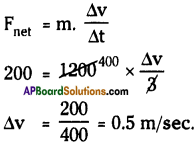Question 7.
What force is required to produce an acceleration of 3 m/sec2 in an object of mass 0.7 kg? (AS 1)
Mass of the object (m) = 0.7 kg.; Acceleration (a) = 3 m/sec²
Force required (F) = ?
F = ma = 0.7 x 3 = 2.1 N

Question 8.
A force acts for 0.2 sec on an object having mass 1.4 kg initially at rest. The force stops to act but the object moves through 4 m in the next 2 seconds, find the magnitude of the force. (AS 1)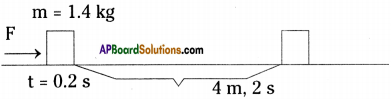Velocity after 0.2 sec v = u + at = 0 + 0.2 a = 0.2a ……….. (1)
After 0.2 s, the body moves with uniform velocity, acceleration is zero, because force is removed.
∴ Velocity v = $$\frac{s}{t}=\frac{4}{2}$$ =2 m/s. ………. (2)
From (1) & (2)
v = 0.2a ⇒ 2 = 0.2a
⇒ a = $$\frac{2}{0.2}$$ = 10 m/s²
∴ Force applied F = ma = 1.4 kg × 10 m/s² = 14 N.Question 9.
An object of mass 5 kg is moving with a velocity of 10 ms-1. A force is applied so that in 15 s, it attains a velocity of 25 ms-1. What is the force applied on the object? (AS 1)
Mass (m) = 5 kg ; Initial velocity (u) = 10 m/s.; Time (t) = 15 s
Final velocity (v) = 25 m/s.
Acceleration a = $$=\frac{v-u}{t}=\frac{25-10}{15}=\frac{15}{15}=1 \mathrm{~m} / \mathrm{s}^{2}$$.
Force applied on the object F = ma = 5 × 1=5 N.

Question 10.
Find the acceleration of body of mass 2 kg from the figures shown. (AS 1)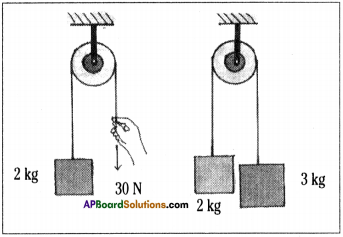1) Force 30 N is acting downwards on weight of (2 × 10) = 20 kg.
The acceleration a = $$\frac{30-20}{2}=\frac{10}{2}$$ = 5 m/s²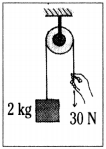2) m1 = 2 kg, m2 = 3 kg.
m2 pulls the body mt with a weight 3 × 10 = 30 N.
∴ Acceleration of m1 = $$\frac{30-20}{3+2}=\frac{10}{5}$$
= 2 m/s².

Question 11.
Take some identical marbles. Make a path or a track keeping your notebooks on either side so as to make a path in which marbles can move. Now use one marble to hit the other marbles. Take two, three marbles and make them to hit the other marbles. What can you explain from your observations? (AS 5)

1. When one marble is hit by another marble, both the marbles move with some velocity.
2. When the marble is hit by two, three marbles, all marbles move with a velocity which is more than in the previous case.
3. As we are hitting with more marbles, the mass increases. So that the net momentum also increases.

Question 12.
A man of mass 30 kg uses a rope to climb which bears only 450 N. What is the maximum acceleration with which he can climb safely? (AS 1, AS 7)
Mass m = 30 kg. ; Force F = 450 N
Acceleration a = ?
F = ma
∴ a = $$\frac{\mathrm{F}}{\mathrm{m}}=\frac{450}{30}$$ =15 m/sec²
∴ The required acceleration =15 m/sec²Question 13.
An vehicle has a mass of 1500 kg. What must be the force between the vehicle and the road if the vehicle is to be stopped with a negative acceleration of 1.7 m/sec²? (AS 1, AS 7)
Mass of the vehicle, m = 1500 kg.; Acceleration (-a) = 1.7 m/sec²
Force, F =?
F = m (-a) = 1500 × (-1.7) = ( – ) 2550 N
∴ The force between the vehicle and road is 2550 N, in the direction opposite to that of the vehicle.

Question 14.
If a fly collides with the windshield of a fast moving bus, is the impact force experienced, same for the fly and the bus? Why? (AS 1, AS 2)
The impact force experienced by the fly will be more, because the mass of fly is negligible when compared to the mass of the bus.

Question 15.
A truck is moving under a hopper with a constant speed of 20 m/sec. Sand falls on the truck at a rate 20 kg/s. What is the force acting on the truck due to falling of sand? (AS 1, AS 7)
Mass of the sand falling on the truck in 1 sec = 20 kg
Constant speed of the truck = 20 m/s
Acceleration in 1 sec, a = $$\frac{\Delta v}{\Delta t}=\frac{20}{1}$$ = 20 m/sec²
Force applied on the truck, F = ma = 20 kg x 20 m/sec² = 400 NQuestion 16.
Two rubber bands stretched to the standard length cause an object to accelerate at 2 m/sec². Suppose another object with twice the mass is pulled by four rubber bands stretched to the standard length. What is the acceleration of the second object? (AS 1)
First object:
Let the force applied by two rubber bands = F1 Newton
Mass of the object = m1 kg; Acceleration a1 = 2 m/sec²
We know F = ma
F1 = m1 × 2
⇒ F1 = 2m1 …….(1)

Second object:
The force applied by 4 rubber bands = 2F1 Newton
Mass of the object = 2m1 kg ; Acceleration a2 =?
We know F = ma
2F1 = 2m1. a2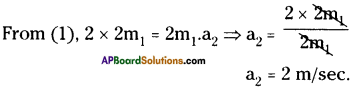∴ Acceleration of the second object = 2 m/sec²

Question 17.
Illustrate an example of each of the three laws of motion. (AS 1)
First law of motion :
A body continues its state of rest or of uniform motion unless a net force acts on it.
Ex:

1. When the bus which is at rest begins to move suddenly, the person standing in the bus falls backward.
2. When you are travelling in bus, the sudden stop of the bus makes you fall forward.

Second law of motion :
The rate of change of momentum of a body is directly proportional to the net force acting on it and it takes place in the direction of net force.
Ex : Place a ball on the veranda and push it gently. Then the ball accelerates from rest. Thus, we can say that force is an action which produces acceleration.

Third law of motion :
If one object exerts a force on the other object, the second object exerts a force on the first one with equal magnitude but in opposite direction.Ex :

1. When birds fly, they push the air downwards with their wings, and the air pushes back the bird in opposite upward direction.
2. When a fish swims in water, the fish pushes the water back and the water pushes the fish with equal force but in opposite direction.
3. A rocket accelerates by expelling gas at high velocity. The reaction force of the gas on the rocket accelerates the rocket in a direction opposite to the expelled gases.

Question 18.
Two ice-skaters initially at rest, push of each other. If one skater whose mass is 60 kg has a velocity of 2 m/s. What is the velocity of other skater whose mass is 40 kg? (AS 1, AS 7)
Mass of first skater m1 = 60 kg.;
Velocity of first skater v1 = 2 m/s.
Mass of second skater m2 = 40 kg.; Velocity of second skater v2 =?
As the two skaters push each other, the resultant momentum will become zero.
The resultant momentum m1v1 + m2v2 = 0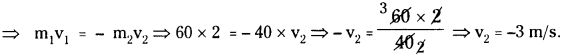∴ Velocity of second skater is 3 m/s, but in the direction opposite to the first skater.

Question 19.
A passenger in moving train tosses a coin which falls behind him. It means that the motion of the train is (AS 7)
a) Accelerated
b) Uniform
c) Retarded
d) Circular motion
a) Accelerated

Question 20.
A horse continues to apply a force in order to move a cart with a constant speed. Explain. (AS 1)

1. The cart moves when the force (in the form of pulling) is applied by the horse.
2. As the horse and cart are moving, the net momentum will be zero at any instance of time.
3. Hence when the horse comes to rest, the cart also comes to rest.
4. To avoid this and to move the cart with a constant speed, the horse must apply force continuously.

Question 21.
A force of 5 N produces an acceleration of 8 m/sec² on a mass m, and an acceleration of 24 m/sec² on a mass m2. What acceleration would the same force provide If both the masses are tied together? (AS 1)
For the first mass (m1)
Force F = 5 N
Acceleration a = 8 m/s²
We know, F = ma
5 = m, . 8
m1= $$\frac{5}{8}$$ kg

For the second mass (m2)
Force F = 5 N
Acceleration a = 24 m/sec²
We know, F = ma
5 = m2.24
m2 = $$\frac{5}{24}$$ kg
When both the masses are tied together and the same force is applied, then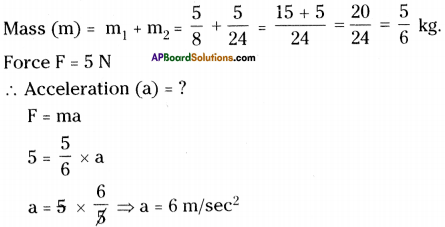Question 22.
A hammer of mass 400 g, moving at 30 m/s, strikes a nail. The nail stops the hammer in a very short time of 0.01 s. What is the force of the nail on the hammer? (AS 1)
Mass of the hammer (m) = 400 g = 0.4 kg. ; Velocity of the hammer (v) = 30 m/s.
Momentum (∆p) = 30 × 0.4 N – s.
The nail stops the hammer with in a time 0.01 s.
∴ ∆t = 0.01 s.
The stopping force of the nail on the hammer.Question 23.
System is shown in figure. Assume there is no friction. Find the acceleration of the liloc-ks-and tension in the string. Take g = 10 m/s² (AS 1)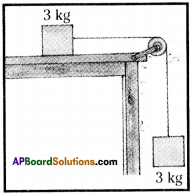m1 = 3 kg, m2 = 3 kg.
1) Acceleration ’a’ and tension T on m1 are shown in figure.
2) Acceleration a and tension T will be as shown in figure.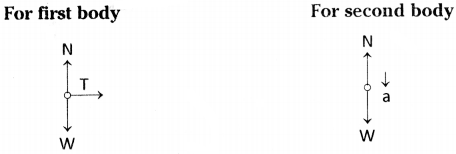Normal force = weight 3s – T = 3a ………… (2)
∴ only tension applied
Tension T = 3 kg × a = 3a
From (1) & (2)
3g – 3a = 3a ⇒ 3g = 6a ⇒ a = $$\frac{3 g}{6}=\frac{3 \times 10}{6}$$ = 5 m/s².
Tension T = 3a = 3 × 5 = 15 N.

Question 24.
Three identical blocks, each of mass 10 kg, are pulled as shown on the hoii ;ontal frictionless surface. If the tension (F) in the rope is 30 N, what is the acceleration oi each block? And what are the tensions in the other ropes? (Neglect the masses of the ropes) (AS 1)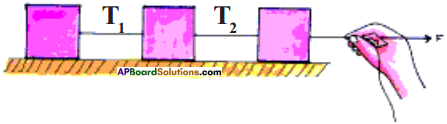Three blocks, each of mass 10 kg are pulled by a rope.
∴ Total mass = 10 + 10 + 10 = 30 kg
Force applied by the rope, F = 30 N
∴ Acceleration of each block, a = $$\frac{F}{m}=\frac{30}{30}$$ = 1 m/sec².

Tension in first rope (T1)
First rope pulls only one block whose mass is 10 kg with an acceleration 1 m/sec².
∴ F = ma = 10 kg × 1 m/sec² = 10 N

Tension in second rope (T2)
Second rope pulls two blocks, each of mass 10 kg.
∴ Total mass = 10 + 10 = 20 kg.
Acceleration, a = 1 m/sec²
Force, F = ma = 20 × 1 = 20 N

Question 25.
A ball of mass’m’ moves perpendicularly to a wall with a speed v, strikes it and rebounds with the same speed in the opposite direction. What is the direction and magnitude of the average force acting on the ball due to the wall? (AS 7)
According to the Newton s third law of motion,
Force exerted by ball on the wall = – (Force exerts by the wall on the ball)
∴ FB.W = – FW.B
Force exerted by ball :
Mass of ball = m, speed = v, FBW = ma = $$\frac{\mathrm{m} \cdot \mathrm{v}}{\mathrm{t}}$$
As the wall is at rest and exerts some force on the ball of mass m, then it moves in the other direction with the same speed.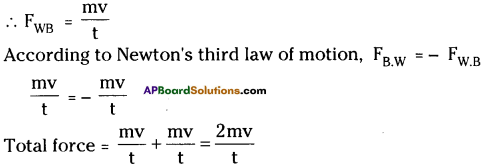Question 26.
Divya observed a horse pulling a cart. She thought that cart also pulls the horse with same force in opposite direction. As per third law of motion, the cart should not move forward. But her observation of moving cart raised some questions in her mind. Can you guess what questions are raised in her mind? (AS 2)

• According to Newton’s third law, when horse pulls a cart, the cart also pulls the horse with same force but in opposite direction. So the cart has to stop. Why is the cart moving?
• What is the effect of friction of ground on the cart and horse?
• Does the cart continue to move even if the horse stops pulling it?
• What makes the cart to move continuously?
• Does it become an isolated system?
• What is the action and reaction in this system?Question 27.
How do you appreciate Galileo’s thought of “any moving body continues in the stale only until some external force acts on it”, which is contradiction to the Aristotle’s belief of “any moving body naturally comes to rest”? (AS 6)

• Science is dynamic.
• All theories can change time to time so that the science and technology will be developed.
• Aristotle’s belief proved to be wrong only by the experiments conducted by Galileo.
• So anybody can challenge the existing theories with proper experimentation.
• Aristotle’s and Galileo’s contradictory thought lead Newton to propose most popular laws of motion.
• Newton’s third law of motion is the basic principle in rocket launching.
• Nowadays we are enjoying the results of satellites launched by rockets.
• Hence comfortable life is the effort on experiments, theories, and calculations made by scientists with a zeal to invent new.

### 9th Class Physical Science 2nd Lesson Laws of Motion InText Questions and Answers

9th Class Physical Science Textbook Page No. 24

Question 1.
Do all the bodies have the same inertia?
The inertia of all bodies is not same. It depends on the mass of the object.

Question 2.
What factors can decide the inertia of a body?
Mass is the factor, which decides the inertia of a body.

9th Class Physical Science Textbook Page No. 26

Question 3.
Is the acceleration increased when net force is increased?
Yes, the acceleration increased, as we increased the net force without changing mass of the object.

9th Class Physical Science Textbook Page No. 29

Question 4.
What do you notice from the readings in the spring balances?
The two spring balances stretch up to a certain limit equally.Question 5.
Are the readings of two spring balances the same?
Yes, the readings of two spring balances are equal.

Question 6.
Are we able to make the spring balances to show different readings by pulling them simultaneously in opposite directions? Why not?
When same force is applied in both the directions, we are unable to make the spring balances to show different readings because the action and reaction are same in magnitude and opposite in direction. When we use two forces with different magnitudes, then the spring balances can show different readings.

9th Class Physical Science Textbook Page No. 30

Question 7.
Does the rocket exert a force on the gas expelled from it?
The rocket also exerts a force on the gas expelled from it.

9th Class Physical Science Textbook Page No. 32

Question 8.
Why does a pole vault jumper land on thick mats of foam?
A thick mat of foam reduces the force of impact of the jumper, so that he doesn’t have any damage to his body.Question 9.
Is it safe to jump on sand rather than a cement floor? Why?

• It is safe to jump on sand rather than a cement floor.
• A soft and more cushioned landing surface provides a greater stopping distance because of the longer time taken to stop.

9th Class Physical Science Textbook Page No. 24

Question 10.
You may have seen the trick where a tablecloth is jerked from a table, leaving the dishes that were on the cloth nearly in their original positions.
a) What do you need to perform this successfully?
We need a table, a cloth and some massive objects to perform this activity. The performer drag the cloth from the table very skillfully.

b) Which cloth should we use? Is it cloth made of thick cotton or thin silk?
We have to use a thin silk cloth to perform this activity.

c) Should the dishes possess large mass or small mass?
The dishes must possess large mass. We should not use lighter objects like plastic cups, etc.

d) Is it better to pull the cloth with a large force or pull it with a gentle and steady force?
The cloth must be pulled with a gentle force, but with a sudden jerk.Question 11.
What is the velocity of a small object that has separated from a rocket moving in free space with velocity 10 km/s?
When a small object is separated from another object which is moving with a certain velocity. The small object also moves with a velocity equal to that of the object from which it is separated. Hence, the speed of the small object is 10 km/s.

9th Class Physical Science Textbook Page No. 27

Question 12.
Observe the following diagram.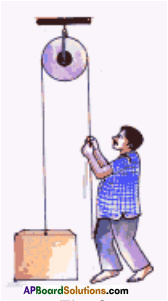What is the upper limit of weight that a strong man of mass 80 kg can lift as shown in figure?
Total force mg = N + T (N = normal force, T = tension)
As the person is standing on the floor, the normal force N = 0.
80 = 0 + T
∴ T = 80
∴ The upper limit of the weight that the person in the figure can lift is 80 kg.

Question 13.
What is the momentum of a ceiling fan when it is rotating?
Ceiling fan when it is rotating, possesses angular momentum.
Angular momentum L = mvr or mr²ω.Question 14.
Is it possible to move in a curved path in the absence of a net force?
A body comes into curved path, when centripetal force real force acts on it. Immediately after coming into curved path, an imaginary force which acts away from the centre i.e., centrifugal force comes into existence. These two forces are equal in magnitude and opposite in direction. Hence the net force is zero. So it is possible to move in a curved path in the absence of a net force.

Question 15.
Prove that the tension throughout the string is uniform when the mass of string is considered to be zero.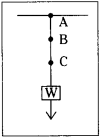Let a body of mass m is suspended through a string. The weight of the object mg acts downwards. Now tension in the string T = mg + msg when ms is the mass of the string.
Here ms is considered as zero.
Hence TA= mg + 0 = mg; TB = mg ; TC = mg ; TD = mg
∴ The tension throughout the string is uniform when the mass of string is considered to be zero.

9th Class Physical Science Textbook Page No. 31

Question 16.
The force exerted by the earth on the ball is 8 N. What is the force on the earth by the ball?

• The force exerted by the earth on the ball is 8 N.
• The force exerted by the ball on the earth is – 8 N.

“According to Newton’s third law, if a body A exerted a force p on another body B, the B exerts a force -p on A, the two forces acting along the same line”.Question 17.
A block is placed on the horizontal surface. There are two forces acting on the block. One, the downward pull of gravity and other a normal force acting on it. Are these forces equal and opposite? Do they form action – reaction pair? Discuss with your friends.
These two forces form action-reaction pair.

Question 18.
Why is it difficult for a fire fighter to hold a hose that ejects large amount of water at high speed?
A large amount of water with high speed ejects from the hose of a fire engine, produces a large force in forward direction. According to action-reaction, the hose moves back with the same force. But the fire fighter has to resist that reaction force. Hence it becomes very difficult for him.

9th Class Physical Science Textbook Page No. 33

Question 19.
A meteorite burns in the atmosphere before it reaches the earth’s surface. What happens to its momentum?
The momentum of the meteorite becomes zero. It doesn’t touch the ground as it burns in the atmosphere. So no mass of the meteorite hits the ground.Question 20.
As you throw a heavy ball upward, is there any change in the normal force on your feet?
The normal force on the feet changes its direction and acts in upward direction. As a result we raise our foot while throwing the ball.

Question 21.
When a coconut falls from a tree and strikes the ground without bouncing. What happens to its momentum?
Its momentum doesn’t change but its impact force will be very less because it is not bouncing.

Question 22.
Air bags are used in’the cars for safety. Why?
When a car hits another vehicle, the air bags immediately comes in between the persons in the car and the wind shield of the car, to prevent damage to life of passengers.

9th Class Physical Science 2nd Lesson Laws of Motion Activities

Activity – 1

Question 1.
Explain the motion of a pen cap kept on a thick paper ring.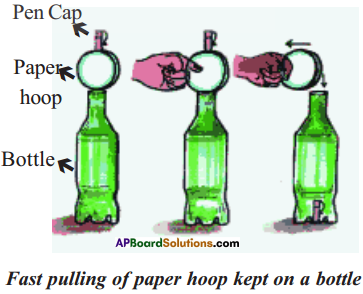1. Make a circular strip from a thick paper.
2. Balance the hoop on the centre of the mouth of the bottle.
3. Now balance a pen cap on the paper hoop aligning it on the centre of the bottle’s mouth.
4. Give the paper hoop a sharp push with your finger as fast as you can.
5. We observe that the pen cap suddenly falls into the bottle.
6. As we push paper hoop, we applied force on the paper hoop. So it changed its state from rest to motion.
7. Pen cap cannot change its state of rest.
8. Due to gravitational force, the pen cap falls into the bottle.

Activity – 2

Question 2.
Explain the motion of the carrom coins hit by a striker.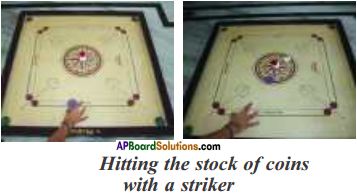1. Make a stack of carrom coins on the carrom board.
2. Give a sharp hit at the bottom of the stack with striker.
3. We can find that the bottom coin will be removed from the stack.
4. The other coins in the stack will slide down.
5. When we apply force on the bottom coin, the coin will move, due to change in the state of rest.
6. The stack of remaining coins does not fall vertically due to inertia.

Activity – 3

Question 3.
Show that the object with larger mass has greater inertia.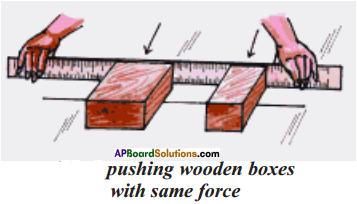1. Take two rectangular wooden blocks with different masses.
2. Place them on a straight line drawn on a floor.
3. Give the same push at the same time to both the blocks with the help of a wooden scale.
4. We observe that the block with small mass will accelerate more and goes farther.
5. The block with large mass accelerates less and moves shorter, due to high inertia.
6. This shows that the bodies of higher mass have high inertia.

Activity – 4

Question 4.
Show that the larger the net force greater the acceleration.

1. Gently push a block of ice on a smooth surface and observe how the object speeds up, in other words how it accelerates.
2. Now increase the net force and observe change in its speed.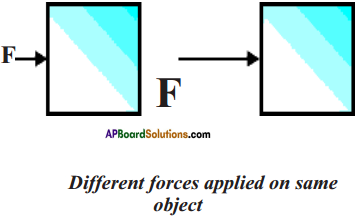Observation : The acceleration increases.

Conclusion : If the net force is larger, then the accelerations greater.

Activity – 5

Question 5.
Show that the larger the mass smaller the acceleration.

1. Apply a force on an ice block.
2. It undergoes some acceleration.
3. Now take a block of ice with greater mass.
4. Then apply almost the same force on the ice block which has greater mass.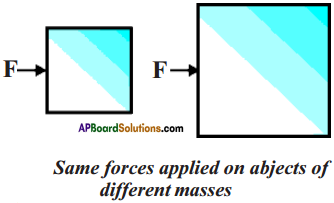Observations :

1. In both cases the object accelerates.
2. But we can observe in the second case, it will not speed up as quickly as before.

Conclusion : If the mass is larger, then the acceleration is smaller.

Activity – 6

Question 6.
Pulling two spring balances.
Let’s take two spring balances of equal calibrations. Connect the two spring balances as shown in figure. Pull the spring balances in opposite directions as shown in figure.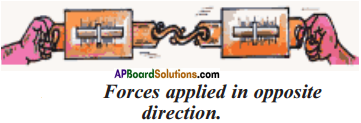Observation :
There is no change in the reading of spring balances. We are not able to make the spring balances to show different readings by pulling them simultaneously in opposite directions.

Conclusion :
According to third law of motion, when an object exerts a force on the other object, the second object also exerts a force on the first one which is equal in magnitude but opposite in direction.

The two opposing forces are known as action and reaction pair. Newton’s third law explains what happens when one object exerts a force on another object.

Activity – 7

Question 7.
Describe the preparation of a balloon rocket. What is the principle involved in it?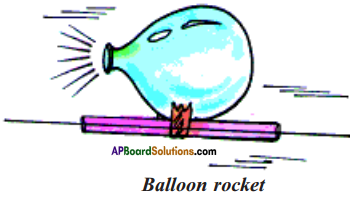Preparation of a balloon rocket :

1. Inflate a balloon and press its neck with fingers to prevent air escaping from it.
2. Pass a thread through a straw and tape the balloon on the straw.
4. Now release air from balloon by removing fingers from the neck of the balloon.
5. The balloon moves like a rocket towards the other end

Principle involved in it:

1. Newton’s third law of motion is the principle.
2. As the air in the balloon moves backward, the balloon moves forward.

Lab Activity

Question 8.
Describe an activity to study the action and reaction forces acting on two different objects.
Aim : To show the action and reaction forces acting on two different objects.

Material required : Test tube, rubber cork cap, Bunsen burner, laboratory stand and thread.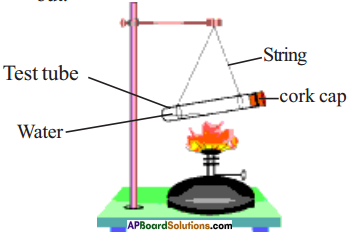Procedure :

1. Take a test tube and put a small amount of water in it.
2. Place a rubber cork cap at its mouth to close it.
3. Now suspend the test tube horizontally to a stand with the help of two strings.
4. Heat the test tube with a bunsen burner until water vapourize and the rubber cork cap blows out.

Observations :

1. Observe the movement of test tube when cork cap blows out.
2. As the cork cap blows out in forward direction, the test tube recoils back.
3. We can observe the velocities of cork cap and recoil of test tube to be same.

Activity – 8

Question 9.
Show that the impulse will be less on a soft and cushioned surface.

• Take two eggs.
• Drop them from a certain height, so that one egg falls on a concrete floor and the other on a cushioned pillow.
• We observe that the egg that falls on a concrete floor will break.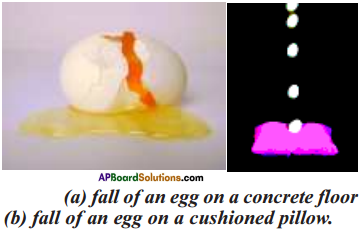• The reason is large force acts on the egg for short interval of time.
∆p = Fnet∆t
• The egg which falls on a cushioned pillow doesn’t break, because a smaller force acts on the egg for a longer time.
∆p= Fnet ∆t
• This shows that the impulse (∆P) will be less on a soft and cushioned surface.

Note : Even if the ∆p is the same in both cases, the magnitude of the net force (Fnet</sub) acting on the egg determines whether the egg will break or not.